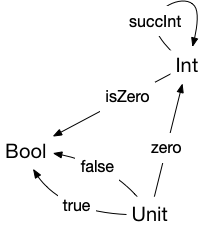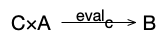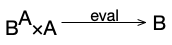# Category Theory Lesson 3: From Arrows to Lambda

Quick personal aside: I haven’t been posting a lot on here lately. I keep wanting to get back to it; but each time I post anything, I’m met by a flurry of crap: general threats, lawsuit threats, attempts to steal various of my accounts, spam to my contacts on linkedin, subscriptions to ashley madison or gay porn sites, etc. It’s pretty demotivating. I shouldn’t let the jerks drive me away from my hobby of writing for this blog!

I started this series of posts by saying that Category Theory was an extremely abstract field of mathematics which was really useful in programming languages and in particular in programming language type systems. We’re finally at one of the first places where you can really see how that’s going to work.

If you program in Scala, you might have encountered curried functions. A curried function is something that’s in-between a one-parameter function and a two parameter function. For a trivial example, we could write a function that adds two integers in its usual form:

  def addInt(x: Int, y: Int): Int = x + y


That’s just a normal two parameter function. Its curried form is slightly different. It’s written:

  def curriedAddInt(x: Int)(y: Int): Int = x +y


The curried version isn’t actually a two parameter function. It’s a shorthand for:

  def realCurrentAddInt(x: Int): (Int => Int) = (y: Int) => x + y


That is: currentAddInt is a function which takes an integer, x, and returns a function which takes one parameter, and adds x to that parameter.

Currying is the operation of taking a two parameter function, and turning it into a one-parameter function that returns another one-parameter function – that is, the general form of converting addInt to realAddInt. It might be easier to read its type: realCurrentAddInt: Int => (Int => Int): It’s a function that takes an int, and returns a new function from int to int.

So what does that have to do with category theory?

One of the ways that category theory applies to programming languages is that types and type theory turn out to be natural categories. Almost any programming language type system is a category. For example, the figure below shows a simple view of a programming language with the types Int, Bool, and Unit. Unit is the initial object, and so all of the primitive constants are defined with arrows from Unit.For the most part, that seems pretty simple: a type T is an object in the programming language category; a function implemented in the language that takes a parameter of type A and returns a value of type B is an arrow from A to B. A multi-parameter function just uses the cartesian product: a function that takes (A, B) and returns a C is an arrow from$A \times B \rightarrow C$.

But how could we write the type of a function like our curried adder? It’s a function from a value to a function. The types in our language are objects in the category. So where’s the object that represents functions from A to B?

As we do often, we’ll start by thinking about some basic concepts from set theory, and then generalize them into categories and arrows. In set theory, we can define the set of functions from$A$ to$B$ as:$B^A={f: A \rightarrow B}$ – that is, as exponentiation of the range of the produced functions.

• There’s a product object$B^A \times A$.
• There’s an arrow from$B^A \times A \rightarrow B$, which we’ll call eval.

In terms of the category of sets, what that means is:

• You can create a pair of a function from$A \rightarrow B$ and an element of$A$.
• There is a function named eval which takes that pair, and returns an instance of$B$.

Like we saw with products, there’s a lot of potential exponential objects$C$ which have the necessary product with$A$, and arrow from that product to$B$. But which one is the ideal exponential? Again, we’re trying to get to the object with thie universal property – the terminal object in the category of pseudo-exponentials. So we use the same pattern as before. For any potential exponential, there’s an arrow from the potential exponential to the actual exponential, and the potential exponential with arrows from every other potential exponential is the exponential.

Let’s start putting that together. A potential exponential$C$ for$B^A$ is an object where the following product and arrow exist:There’s an instance of that pattern for the real exponential:We can create a category of these potential exponentials. In that category, there will be an arrow from every potential exponential to the real exponential. Each of the potential exponentials has the necessary property of an exponential – that product and eval arrow above – but they also have other properties.

In that category of potential exponentials of$B^A$, there’s an arrow from an object$X$ to an object$Y$ if the following conditions hold in the base category:

• There is an arrow$\text{curry}(x,y): X \rightarrow Y$ in the base category.
• There is an arrow$\text{curry}(x,y)\times id_A: X\times A \rightarrow Y\times A$
•$\text{eval}_y(\text{curry}(x,y)\times id_A=\text{eval}_y y$

It’s easiest to understand that by looking at what it means in Set:

• We’ve got sets$X$ and$Y$, which we believe are potential exponents.
•$X$ has a function$\text{eval}_x: X \times A \rightarrow B$.
•$Y$ has a function$\text{eval}_y: Y \times A \rightarrow B$.
• There’s a function$\text{curry}: X \rightarrow Y$ which converts a value of$X$ to a value of$Y$, and a corresponding function$\text{curry}(\cdot)\times\text{id}_A: X\times A \rightarrow Y\times A$, which given a pair$(x, a) \in X\times A$ transforms it into a pair$(y, a) \in Y\times A$, where evaluating$\text{eval}_x(x, a)=\text{eval}_y(\text{curry}(x, a))$. In other words, if we restrict the inputs to$Y$ to be effectively the same as the inputs to$X$, then the two eval functions do the same thing. (Why do I say restrict? Because$\text{eval}_y$ might have a larger domain than the range of$X$, but these rules won’t capture that.)

An arrow in the category of potential products is a pair of two arrows in the base category:  one from$C \rightarrow B^A$, and one from$C\times A \rightarrow B^A \times A$ . Since the two arrows are deeply related (they’re one arrow in the category of potential exponentials), we’ll call them$\text{curry}(g)$ and$\text{curry}(g)\times id_A$. (Note that we’re not really taking the product of an arrow here: we haven’t talked about anything like taking products of arrows! All we’re doing is giving the arrow a name that helps us understand it. The name makes it clear that we’re not touching the right-hand component of the product.)

Since the exponential is the terminal, which means that that pair of curry arrows must exist for every potential exponential to the true exponential. So the exponential object is the unique (up to isomorphism) object for which the following is true:

• There’s an arrow$\text{eval}: B^A \times A \rightarrow A$. Since$B^A$ is the type of functions from$A$ to$B$,$\text{eval}$ represents the application of one of those functions to a value of type$A$ to produce a result of type$B$.• For each two-parameter function$g:C\times A\rightarrow B$, there is a unique function (arrow)$\text{curry}(g)$ that makes the following diagram commute

Now, how does all this relate to what we understand as currying?

It shows us that in category theory we can have an object that is effectively represents a function type in the same category as the object that represents the type of values it operates on, and you can capture the notion of applying values of that function type onto values of their parameter type using an arrow.

As I said before: not every category has a structure that can support exponentiation. The examples of this aren’t particularly easy to follow. The easiest one I’ve found is Top the category of topological spaces. In Top, the exponent doesn’t exist for many objects. Objects in Top are topological spaces, and arrows are continuous functions between them. For any two objects in Top, you can create the necessary objects for the exponential. But for many topological spaces, the required arrows don’t exist. The functions that they correspond to exist in Set, but they’re not continuous – and so they aren’t arrows in Top. (The strict requirement is that for an exponential$X^Y$ to exist,$Y$ must be a locally compact Hausdorff space. What that means is well beyond the scope of this!)

### Cartesian Closed Categories

If you have a category$C$, and for every pair of objects$A$ and$B$ in the category$C$, there exists an exponential object$B^A \in C$, then we’ll say that$C$ has exponentiation. Similarly, if for every pair of objects$A, B \in Ob(C)$, there exists a product object$A\times B$, we say that the category has products.

There’s a special kind of category, called a cartesian closed category, which is a category  where:

1. Every pair of objects has both product and exponent objects; and
2. Which has at least one terminal object. (Remember that terminals are something like singletons, and so they work as a way of capturing the notion of being a single element of an object; so this requirement basically says that the category has at least one value that “functions” can be applied to.)

That may seem like a very arbitrary set of rules: what’s so important about having all products, exponents, and a terminal object?

It means that we have a category which can model types, functions, and function application. Lambda calculus proves that that’s all you need to model computation. Closed cartesian categories are, basically, a formal model of a computing system! Any cartesian closed category is a model for a simply typed$\lambda$-calculus; and$\lambda$-calculus is something known as the internal language of a cartesian closed category.

What “internal language” means formally is complicated, but in simple terms: you can take any computation in lambda calculus, and perform the computation by chasing arrows in a category diagram of a closed cartesian category that includes the values of that calculus. Alternatively, every computation step that you perform evaluating a$\lambda$-calculus expression corresponds to an arrow in a CCC.

### References

For this post, I’ve made heavy use of: